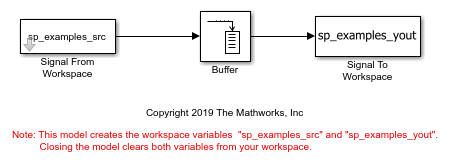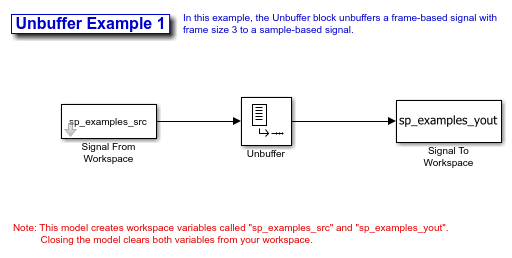# rebuffer_delay

Number of samples of delay introduced by buffering and unbuffering operations

## Syntax

```d = rebuffer_delay(f,n,v) d = rebuffer_delay(f,n,v,'mode') ```

## Description

`d = rebuffer_delay(f,n,v)` returns the delay, in samples, introduced by the Buffer or Unbuffer block in multitasking operations.

`d = rebuffer_delay(f,n,v,'mode')` returns the delay, in samples, introduced by the Buffer or Unbuffer block in the specified tasking mode.

## Input Arguments

 `f` Frame size of the input to the Buffer or Unbuffer block. `n` Size of the output buffer. Specify one of the following: The value of the Output buffer size parameter, if you are computing the delay introduced by a Buffer block.`1`, if you are computing the delay introduced by an Unbuffer block. `v` Amount of buffer overlap. Specify one of the following: The value of the Buffer overlap parameter, if you are computing the delay introduced by a Buffer block.`0`, if you are computing the delay introduced by an Unbuffer block. `'mode'` The tasking mode of the model. Specify one of the following options: `'singletasking'``'multitasking'` Default: `'multitasking'`

## Examples

collapse all

Compute the delay introduced by a Buffer block in a multitasking model.

Open the `ex_buffer_tut4` model.Double-click the Buffer block to open the block mask. Verify that you have the following settings:

• Output buffer size = 3

• Buffer overlap = 1

• Initial conditions = 0

Based on these settings, two of the required inputs to the `rebuffer_delay` function are as follows:

• n = 3

• v = 1

To determine the frame size of the input signal to the Buffer block, open the Signal From Workspace block mask. Verify that you have the following settings:

• Signal = `sp_examples_src`

• Sample time = 1

• Samples per frame = 4

Because Samples per frame = 4, you know the `f` input to the `rebuffer_delay` function is 4. After you verify the values of all the inputs to the `rebuffer_delay` function, determine the delay that the Buffer block introduces in this multitasking model. To do so, type the following at the MATLAB command line:

```rebuffer_delay(4,3,1) ```
```ans = 8 ```

Compute the delay introduced by an Unbuffer block in a multitasking model.

Open the `ex_unbuffer_ref1` model.To determine the frame size of the input to the Buffer block, open the Signal From Workspace block mask by double-clicking the block in your model. Verify that you have the following settings:

• Signal = `sp_examples_src`

• Sample time = 1

• Samples per frame = 3

Because Samples per frame = 3, you know the `f` input to the `rebuffer_delay` function is 3. Use the `rebuffer_delay` function to determine the amount of delay that the Unbuffer block introduces in this multitasking model. To compute the delay introduced by the Unbuffer block, use f = 3, n = 1 and v = 0.

```rebuffer_delay(3,1,0) ```
```ans = 3 ```

collapse all

When you run a model in `MultiTasking` mode, Simulink® processes groups of blocks with the same execution priority through each stage of simulation based on task priority. Multitasking mode helps to create valid models of real-world multitasking systems, where sections of your model represent concurrent tasks. The Treat each discrete rate as a separate task parameter on the Solver (Simulink) pane of the Configuration Parameters dialog box controls this setting.

When you run a model in `SingleTasking` mode, Simulink processes all blocks through each stage of simulation together. The Treat each discrete rate as a separate task parameter on the Solver (Simulink) pane of the Configuration Parameters dialog box controls this setting.